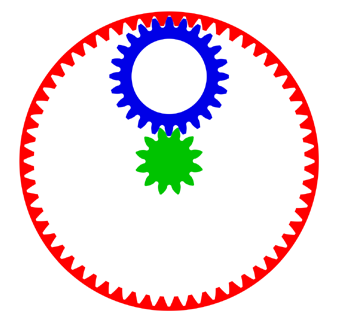Home  •  Tutorials  •  Calculators  •  3D Models  •  About  •  Contact

Internal/External Gear Calculator

The following online calculator computes the basic dimensions and tooth profiles of a bevel gear pair (pinion and gear) based on their number of teeth and angle between the shaft axes.An internal gear is a gear with its teeth cut in the internal surface of a cylinder and meshes with regular, or external, gears.

This online calculator generates the tooth profile equations and other parameters for an internal/external gear pair shown on this picture in red and blue. It can also be used to model a stand-alone center gear shown in green. In a planetary mechanism, the outer gear (red) is called the ring, the center gear (green) is called the run, and the gear meshing with both the sun and ring (blue) is called a planet gear.

Let's denote the number of teeth in the ring gear as R, the number of teeth in the sun gear as S and the number of teeth in the planet gear as P. The three numbers must be chosen to satisfy the following equation:

R = 2 x P + S

In the depicted mechanism, R = 60, P = 24 and S = 12.

If the input shaft is connected to the sun gear and output shaft to an arm on which the planet gear is mounted (referred to as the carrier), the gear ratio is calculated as:

u = (R / S) + 1

In the depicted mechanism, the gear ratio is 1:6.

If the sun gear has 12 teeth or fewer, a positive correction, called "profile shift", needs to be applied to the gear's tooth profile. The same profile shift value needs to be applied to the ring gear as well. In our example, the value of 0.5 can be used. For more information, see Tutorial #04.

Important: In a planetary mechanism, the number of teeth in the sun and ring gears must be divisible by the number of planet gears. Typically, there are 3 planet gears, but sometimes there are 2, 4 or more. The 60/24/12 combination used in our example will work with 2, 3, or 4 planet gears. However, a 60/20/20 combination will work with 2 or 4 planet gears but won't work with 3, as 20 is not divisible by 3.

Basic Dimensions
ParameterSymbolExternalInternal
Modulem
Pressure angleα
Number of teethz
Profile shiftx
Angle between teeth (360° / z)ε
Center distancea